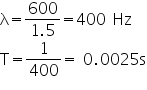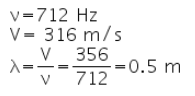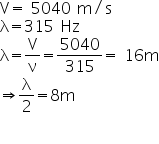Test: Characteristics Of Sound Waves

# Test: Characteristics Of Sound Waves - Class 9

Test Description

## 20 Questions MCQ Test Science Class 9 - Test: Characteristics Of Sound Waves

Test: Characteristics Of Sound Waves for Class 9 2023 is part of Science Class 9 preparation. The Test: Characteristics Of Sound Waves questions and answers have been prepared according to the Class 9 exam syllabus.The Test: Characteristics Of Sound Waves MCQs are made for Class 9 2023 Exam. Find important definitions, questions, notes, meanings, examples, exercises, MCQs and online tests for Test: Characteristics Of Sound Waves below.
Solutions of Test: Characteristics Of Sound Waves questions in English are available as part of our Science Class 9 for Class 9 & Test: Characteristics Of Sound Waves solutions in Hindi for Science Class 9 course. Download more important topics, notes, lectures and mock test series for Class 9 Exam by signing up for free. Attempt Test: Characteristics Of Sound Waves | 20 questions in 20 minutes | Mock test for Class 9 preparation | Free important questions MCQ to study Science Class 9 for Class 9 Exam | Download free PDF with solutions
 1 Crore+ students have signed up on EduRev. Have you?
Test: Characteristics Of Sound Waves - Question 1

### Which of the following correctly relates the wavelength λ, frequency f and velocity v of sound?​

Detailed Solution for Test: Characteristics Of Sound Waves - Question 1

We know that, T = 1 / f
Where, f is the frequency, T is time period
Also, Speed  =  Distance / Time taken
Speed of wave is it's velocity v, and Distance of wave is it's wavelength lemda.
Substituting these into the equation.
=>  v = lemda / T
=>    v  = lemda  f
As,  T =  1/ f
Therefore,  v = f lemda

Test: Characteristics Of Sound Waves - Question 2

### The wavelength of the sound produced by a tuning fork of frequency 450 Hz which is set to vibrate in the air (take the velocity of sound in air as 320 m/s) is

Detailed Solution for Test: Characteristics Of Sound Waves - Question 2

Wavelength = speed of air (v) / frequency (ν)

Wavelength = 320/ 450= 0.711 m

Test: Characteristics Of Sound Waves - Question 3

### A siren has 16 holes and is making 960 revolutions per minute. The frequency of the sound produced by the siren is:

Detailed Solution for Test: Characteristics Of Sound Waves - Question 3

Number of holes h = 16
Revolutions per minute = 960

Revolutions per second =960/60 =16

frequency = no. of holes x revolutions per sec

= 16 x16 =256 Hz

Test: Characteristics Of Sound Waves - Question 4

SI unit of frequency is

Detailed Solution for Test: Characteristics Of Sound Waves - Question 4

The  hertz (symbol Hz) is the SI unit of frequency defined as the number of cycles per second of a periodic phenomenon.

This unit is named after a German physicist Heinrich Hertz.

Test: Characteristics Of Sound Waves - Question 5

Arrange in increasing order – Speed of sound in steel, in distilled water, in sea water and in oxygen.

Detailed Solution for Test: Characteristics Of Sound Waves - Question 5

The speed of sound decreases as we go from solid to gaseous state because the density of particles decreases from solid to gaseous.

Test: Characteristics Of Sound Waves - Question 6

A top makes 16 rotations about its own axis within 0.4 seconds, its frequency is:

Detailed Solution for Test: Characteristics Of Sound Waves - Question 6

In 0.4 sec=16 rotations,

so in, 1 sec = 16/0.4

=160/4

=40 rotations

Test: Characteristics Of Sound Waves - Question 7

On which quantity does the pitch of the sound depend?

Detailed Solution for Test: Characteristics Of Sound Waves - Question 7

The pitch of sound depends on the frequency of vibration. The higher the frequency the more shrill is the sound and vice versa.

Test: Characteristics Of Sound Waves - Question 8

What is the difference between a low pitched and high pitched sound?

Detailed Solution for Test: Characteristics Of Sound Waves - Question 8

High pitch sound is a sound which has a high frequency. usually shrill sounds have a high pitch. for ex. a whistle has high pitch.

low pitch sounds are sounds which have low frequency rate. for ex. horse voice.

loud sounds have a high amplitude. for ex. a mega phone gives sound of high amplitude.

soft sounds are sounds which have low amplitude. for ex. a birds chirping has low amplitude.

Test: Characteristics Of Sound Waves - Question 9

A compression is a region of

Detailed Solution for Test: Characteristics Of Sound Waves - Question 9

Compression is the region of high pressure and rarefaction is the region of low pressure. Higher the pressure in a region, higher is the number of particles per unit volume and hence higher is the density of the medium. So, a sound wave propagates through a medium as the variation in its pressure or density.

Test: Characteristics Of Sound Waves - Question 10

Sound from a flute and sound from a harmonica played by passing air through musical instrument, at same time and same distance from ear and traveling through same medium air, they differ in sounds due to difference in

Detailed Solution for Test: Characteristics Of Sound Waves - Question 10

Pitch of sound is how brain interprets the frequency of the emitted sound. In harmonica, vibration of particles is more at the source, and hence more frequency. Therefore, it has higher pitch. Thus, harmonica sound appears different than sound of flute

Test: Characteristics Of Sound Waves - Question 11

A stationary rail engine produces whistle with a frequency 2600 Hz. A person standing at a distance of 550 m from engine will listen ___________ no. of waves within one minute.​

Detailed Solution for Test: Characteristics Of Sound Waves - Question 11

Given:
Frequency of the whistle = 2600 Hz
Distance from the engine to the person = 550 m
To find the number of waves heard by the person in one minute, we need to convert the frequency from Hz to waves per minute.
Step 1: Convert frequency to waves per second:
- The frequency of the whistle is given in Hz, which represents the number of waves produced per second.
- Therefore, the number of waves per second can be calculated as follows:
Waves per second = Frequency (Hz) = 2600 Hz
Step 2: Convert waves per second to waves per minute:
- Since we need to find the number of waves heard in one minute, we need to convert waves per second to waves per minute.
- There are 60 seconds in a minute, so the conversion can be done by multiplying waves per second by 60:
Waves per minute = Waves per second x 60 = 2600 Hz x 60 = 156000 waves/minute
Therefore, the person standing at a distance of 550 m from the engine will listen to 156 x 10^3 waves within one minute.
Hence, the answer is option A: 156 x 10^3.
Test: Characteristics Of Sound Waves - Question 12

If we increase the temperature of air from 0°C to 22°C, then the speed of sound would

Detailed Solution for Test: Characteristics Of Sound Waves - Question 12

When the temperature increases then the kinetic energy of the particles also increases as a result they move more faster and so the energy is more fastly transferred through the molecules of the medium.

Test: Characteristics Of Sound Waves - Question 13

Compressions are the regions where

Detailed Solution for Test: Characteristics Of Sound Waves - Question 13

A compression is a region in a longitudinal wave where the particles are closest together. A rarefaction is a region in a longitudinal wave where the particles are furthest apart.

Test: Characteristics Of Sound Waves - Question 14

By counting the number of vibrations of a tuning fork per second, __________ can be found.

Detailed Solution for Test: Characteristics Of Sound Waves - Question 14
Counting Vibrations of a Tuning Fork
The number of vibrations of a tuning fork per second can provide information about the frequency of the wave. Here's a detailed explanation:
Frequency of a Wave:
- The frequency of a wave refers to the number of complete vibrations or cycles that occur in one second.
- It is typically measured in Hertz (Hz) or cycles per second.
- The frequency determines the pitch of a sound wave, with higher frequencies corresponding to higher pitches and lower frequencies corresponding to lower pitches.
Counting Vibrations:
- A tuning fork is a device that produces a specific pitch or frequency when struck.
- By counting the number of vibrations the tuning fork makes in one second, we can determine the frequency of the wave it produces.
- This can be done using various methods, such as using a stopwatch or a device specifically designed to measure frequency.
Importance of Frequency:
- The frequency of a sound wave is one of its fundamental characteristics.
- It helps us differentiate between different sounds and determine their pitch.
- By measuring the frequency of a tuning fork, we can compare it to the expected frequency and determine if it is in tune or not.
In conclusion, by counting the number of vibrations of a tuning fork per second, we can find the frequency of the wave it produces. This information is important for understanding the pitch of the sound and for tuning musical instruments.
Test: Characteristics Of Sound Waves - Question 15

A bell vibrates 600 times within 3/2 seconds, its frequency and time period will be:

Detailed Solution for Test: Characteristics Of Sound Waves - Question 15Test: Characteristics Of Sound Waves - Question 16

A swing has a time period of 1.4 seconds. The no. of oscillations made by it within 1 minute 24 seconds will be:​

Detailed Solution for Test: Characteristics Of Sound Waves - Question 16

Oscillation  = time / time period
⇒ 84 second / 1.4 second
⇒ 60

Test: Characteristics Of Sound Waves - Question 17

Choose one of the following, on which the frequency of a vibrating body does not depend.

Detailed Solution for Test: Characteristics Of Sound Waves - Question 17

When waves travel from one medium to another the frequency never changes. As waves travel into the denser medium, they slow down & wavelength decreases. Part of the wave travels faster for longer causing the wave to turn. The wave is slower but the wavelength is shorter means frequency remains the same.

Test: Characteristics Of Sound Waves - Question 18

A baby cries with a frequency 712 Hz, if the speed of sound in air is 356m/s, the wavelength of sound is:

Detailed Solution for Test: Characteristics Of Sound Waves - Question 18Test: Characteristics Of Sound Waves - Question 19

A progressive transverse wave has a speed of 5040 m/s, its frequency is 315 Hz, then the distance between a crest and an immediate trough is:

Detailed Solution for Test: Characteristics Of Sound Waves - Question 19Test: Characteristics Of Sound Waves - Question 20

Sound of single frequency is called

Detailed Solution for Test: Characteristics Of Sound Waves - Question 20

The only sound which consists of a single frequency is the pure SINE TONE such as produced by a sine wave OSCILLATOR or approximated by a tuning fork. All other sounds are complex, consisting of a number of frequencies of greater or lesser intensity. The frequency content of a sound is its SPECTRUM.

## Science Class 9

66 videos|352 docs|97 tests
Information about Test: Characteristics Of Sound Waves Page
In this test you can find the Exam questions for Test: Characteristics Of Sound Waves solved & explained in the simplest way possible. Besides giving Questions and answers for Test: Characteristics Of Sound Waves, EduRev gives you an ample number of Online tests for practice

## Science Class 9

66 videos|352 docs|97 tests